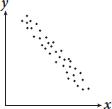# SAT Math Multiple Choice Question 851: Answer and Explanation

### Test Information

Question: 851

11. Which of the following scatterplots could be modeled by the equation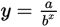, where a and b are constants such that a > 1 and x < –1?

• A.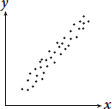• B.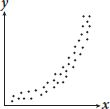• C.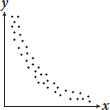• D.